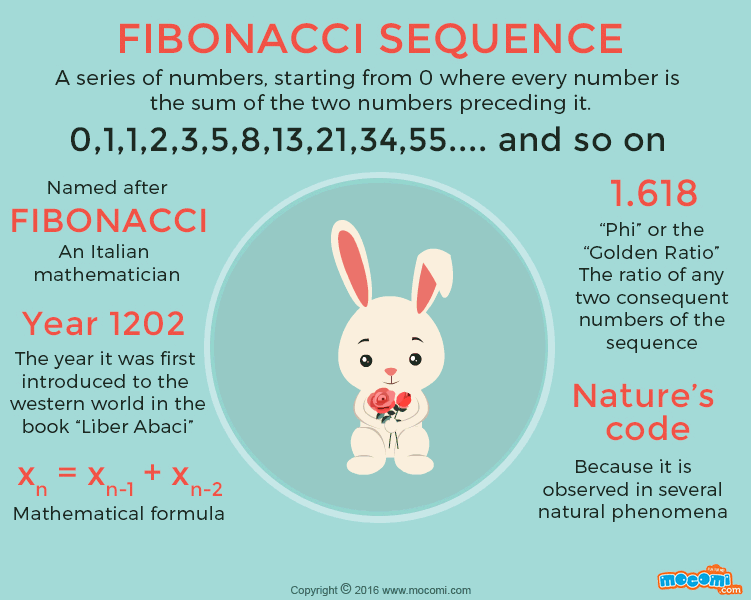• 0
• 0
• 0
• 0
• Embed CodePrevious Article
Next Article

# What is Fibonacci Series?

Gifographic | 7-14 yrs | Reading Pod, Animation

If you’ve read the book “The Da Vinci Code” then you already know about the Fibonacci sequence. This intriguing sequence of numbers is an integral part of what is known as “the golden ratio” which is said to be the ratio in which many natural phenomena occur. Be it a tree branching out or a cluster of stars, the golden ratio can be observed in most naturally occurring phenomena. So let’s find out some more about this mysterious series of numbers.

## What is the Fibonacci Sequence?

The Fibonacci sequence is a series of numbers starting from 0 where every number is the sum of the two numbers preceding it. Thus, the sequence goes 0,1, 2, 3, 5, 8, 13, 21, 34, and so on. The mathematical equation that represents this sequence is  xn = xn-1 + xn-2.

## Who invented Fibonacci Sequence?

The sequence is named after Fibonacci, an Italian mathematician who was also known as Leonardo of Pisa, or Leonardo Pisano. He was a man who traveled widely and traded extensively. As knowledge of mathematics was important to traders, his interest was cultivated in his youth. The Fibonacci numbers were first introduced to the Western World through his book Liber Abaci in 1202. However, the discovery of this sequence precedes Fibonacci; it was discovered and described as “Virahanka” in Indian mathematic scriptures.

### Fibonacci and the Rabbits Problem

Fibonacci first noticed this sequence when he pondered the question of rabbit breeding. The question he pondered was: Beginning with a male and female rabbit, how many pairs of rabbits could be born in a year? After making certain assumptions he deduced a certain pattern to the breeding of rabbits. The pattern followed the Fibonacci sequence. If you’re curious, the answer he came to was 233 pairs of rabbits at the end of a year.

## Why is Fibonacci called as Nature’s Code?

The Fibonacci sequence has a greater significance than simply answering hypothetical rabbit breeding questions. This mysterious sequence appears all around us in nature. The petals of a flower, the seeds of fruits, rows of seeds on a sun flower or the lobes of pinecones and even the spirals on a shell develop or add up to the Fibonacci numbers.

### The Golden Ratio: Phi

Why, you ask? Simply because nature always follows the most efficient way of doing things. And that’s why the Fibonacci numbers are also called nature’s code. Another interesting thing of note is that when you divide any Fibonacci number (say 8), with its preceding number (say 5), the result is very close to 1.618, which is also called “Phi”. Go ahead, pick some more numbers off the sequence and try it! Considering all the beautiful wonders of nature created in this ratio, it was deemed the Golden Ratio. “Phi” is also believed to have been used in ancient Greece to denote the ratio of physical perfection.

The beauty of nature is guided by Mathematics. Doesn’t that make math just a little bit more interesting, if not a whole lot?

•
189
Shares
•
•
•
• 189
•
•
•

## RECOMMENDED ARTICLES

1.Niranjan